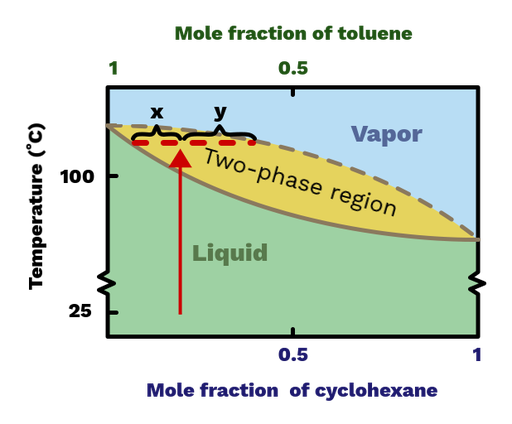The lever rule is a formula used to determine the ratio between two phases of a phase diagram. If we heat a liquid mixture with 0.2 mole fraction (20 mol%) of cyclohexane and 0.8 mole fraction (80 mol%) of toluene to 109 °C, we get a mixture of liquid and vapor. A Lever line is then a horizontal line across the diagram at this temperature, as we see in Figure 1. We calculate the mole fraction of vapor (ωV) and liquid (ωL) in the following way, where x and y are defined in the figure:

ωL = y / (y + x) = 0.6 mole fraction

ωV = x / (y + x) = 0.4 mole fractionFigure 1: Diagram breaking down how you can use the lever rule to interpret the contents of your mixture from a boiling point-composition chart.

In addition to calculating the fraction of each phase, we can use the Lever line to find the composition of the liquid and vapor phases. By drawing a vertical line from the points where the Lever line intersects the boundaries to the liquid and vapor phase, we can find the composition of those phases, respectively. With the help of the Lever rule, we can quantify and predict the number of theoretical plates needed to achieve the desired separation.